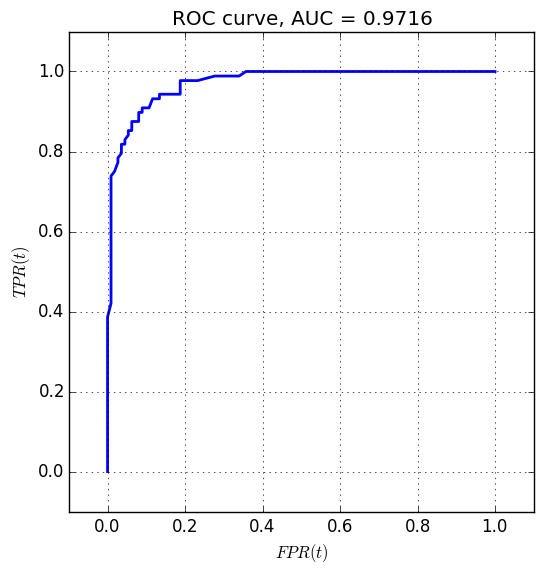This short post is a numerical example (with Python) of the concepts of the ROC curve and AUC score introduced in this post using the logistic regression example introduced in theory here and numerically with Python here.

First let’s import the usual libraries and set some parameters:

Next, we generate testing data which will be used to generate the ROC curve and AUC score. We also compute the logistic regression model’s prediction for this testing data.

Next, we generate $TP(t), TN(t), FP(t), FN(t)$ using a discrete number of thresholds. From these we generate $FPR(t)$, $TPR(t)$, and the ROC curve.

Finally, we compute the AUC score for the ROC curve using the trapezoidal method, and show the plot.

This produces: